# PSEB 4th Class Maths Solutions Chapter 5 Measurement Ex 5.7

Punjab State Board PSEB 4th Class Maths Book Solutions Chapter 5 Measurement Ex 5.7 Textbook Exercise Questions and Answers.

## PSEB Solutions for Class 4 Maths Chapter 5 Measurement Ex 5.7

Question 1.
(a) 8 kg 450 gm + 1 kg 210 gm
Solution: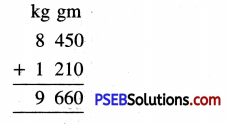(b) 5 kg 675 gm + 2 kg 205 gm
Solution: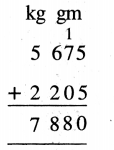(c) 3 kg 225 gm + 7 kg 527 gm
Solution: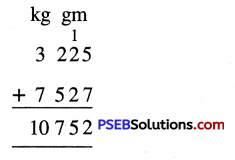(d) 3 kg 050 gm + 1 kg 400 gm
Solution: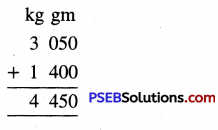(e) 9 kg 100 gm + 5 kg 075 gm
Solution: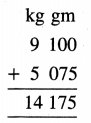(f) 4 kg 650 gm + 6 kg 275 gm
Solution: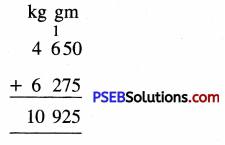Question 2.
Subtract:

(a) 5 kg 845 gm — 2 kg 525 gm
Solution: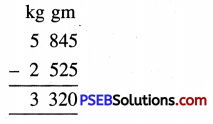(b) 9 kg 605 gm — 6 kg 275 gm
Solution: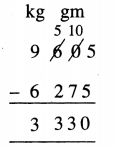(c) 8 kg 360 gm — 3 kg 150 gm
Solution: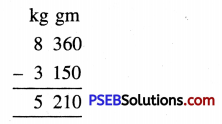(d) 6 kg 320 gm— 4 kg 175 gm
Solution: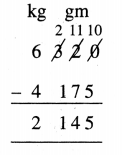(e) 4 kg 500 gm — 1 kg 250 gin
Solution: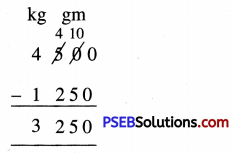(f) 7 kg 425 gm — 6 kg 280 gm
Solution: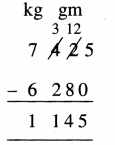Question 3.
Dilpreet bought 5 kg 500 gm of potatoes and 2 kg 250 gm cauliflower. How much vegetables did he buy ?
Solution:
Dilpreet bought quantity of potatoes = 5 kg 500 gm
Dilpreet bought quantity of cauliflower = 2 kg 250 gm
Total quantity of vegetables bought = 7 kg 750 gm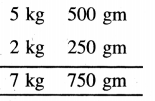Question 4.
Harjot’s weight is 20 kg 500 gm less than that of his brother. If his brother’s weight is 62 kg 750 gm. Find the weight of Harjot.
Solution:
Weight of Harjot’s Brother = 62 kg 750 gm
Harjot’s weight is less than his brother’s weight by = -20 kg 500 gm
Weight of Haijot = 42 kg 250 gm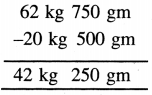Question 5.
A dealer bought 80 kg 500 gm apples. He sold 4 kg 400 gm. What is the weight of remaining apples ?
Solution:
Quantity of apples bought = 80 kg 500 gm
Quantity of apples sold = 4 kg 400 gm
Weight of remaining apples = 76 kg 100 gm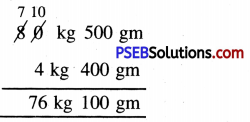Question 6.
An NGO distributed roasted gram (Chana) packets in flood affected area. The weight of every packet is 2 kg. NGO distributed 450 packets. How many kgs of packets they distributed ?
Solution:
Weight of each packet = 2 kg
Total number of packets distributed = 450
Total weight of packets distributed = 2 kg × 450
= 900 kg.